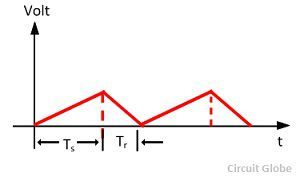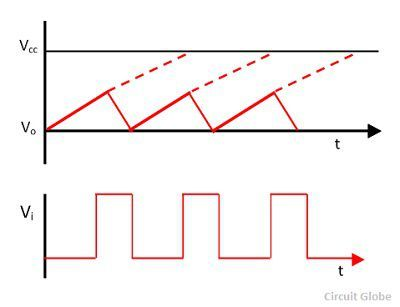# Time Base Generator

Definition: The generator which is used for generating the linear variable voltage concerning time is known as the time base generator. These waves are used in cathode ray tube for deflecting the beam in a horizontal direction. The time base generator is also used in a radar system for determining the range of the target.

The oscilloscope uses a waveform that varies with the time. For accurately reproducing the waveform, it must have a constant horizontal velocity. The velocity operates with the deflecting voltage increases linearly with the time. The voltage having such characteristic is called ramp voltage. The sawtooth waveform produces when the waveform rapidly tends to zero.The figure of the sawtooth waveform is shown above. The sweep time Ts is the time in which the voltage rises linearly. The Ts moves left to right across the CRT screen. The restoring or retrace time Tr is the time required by the waveform to return to its initial position. The restoring time is always less than the sweep time. The sawtooth waveform is obtained when the retrace time becomes zero.

The sweep rate of the sawtooth waves depends on the capacitor used in the circuit. The sweep rate is controlled by the resistor placed in the circuit. Consider a simple sweep circuit in which the capacitor C charges through resistor R. The capacitor discharges periodically through the transistor Q1

### Time Base Generator Circuit

The variable resistor R. charges the capacitor in the circuit. The capacitor is discharged through the transistor Q1. The charge and the discharges of the capacitor generate the waveform shown in the figure below.The transistor Q1 becomes turn on by the help of the input signal Vi. The transistor provides the low resistance through which the capacitor becomes discharges.If the transistor is not turned on the capacitor will charge exponentially to the supply voltage Vcc according to the equation.Where, Vo = instantaneous voltage across the capacitor at time t
Vcc = supply voltage
T= time of interest
R = value of series resistor
C = Value of capacitor

The instantaneous voltage and the supply voltage is measured in volts, the time is measured in second, the resistance is measured in ohm, and the capacitor is measured in Farad.